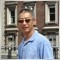# Why can't use {double d=40; d%=4;} returns err. How to work around this, since I need use double?434

Thx.89

'%' - remainder operator is to be applied to integer values only

So, change it to {int d=40, d%=4;}.1674

diostar:
Thx.
Er, just use MathMod()? d = MathMod(d, 4)434

jjc:
Er, just use MathMod()? d = MathMod(d, 4)

Thx!